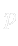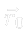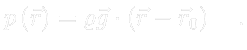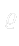## Hydrostatic pressure

Let us consider an incompressible fluid at rest in the presence of a homogeneous gravitational field so it is supported by walls of some, possibly open, vessel. Inside a volume of the fluid there is the well known hydrostatic pressure, let us denote it by. Assume the fluid has one free3.5 part of its boundary, i.e. one part of its surface forms a level, i.e. plane or its part. Let us denote bya position vector3.6 of an arbitrary point of this plane. Hence forholdsNotice the relation () is valid inside the volume of the fluid only. If we need to extend its domain, it is necessary to assure zero values foroutside of the volume of the fluid. For instance, this is satisfied naturally, if we considera constant scalar field but vanishing outside the volume of the fluid.